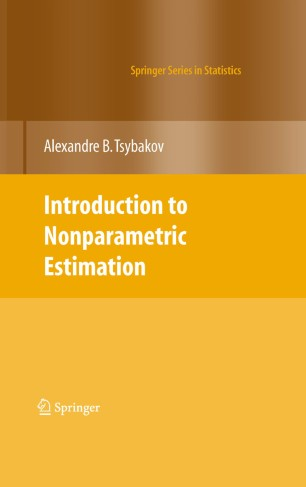# Introduction to Nonparametric Estimation

• Alexandre B. TsybakovPart of the Springer Series in Statistics book series (SSS)

1. Front Matter
Pages 1-10
2. Alexandre B. Tsybakov
Pages 1-76
3. Alexandre B. Tsybakov
Pages 77-135
4. Alexandre B. Tsybakov
Pages 137-190
5. Back Matter
Pages 1-22

### Introduction

Methods of nonparametric estimation are located at the core of modern statistical science. The aim of this book is to give a short but mathematically self-contained introduction to the theory of nonparametric estimation. The emphasis is on the construction of optimal estimators; therefore the concepts of minimax optimality and adaptivity, as well as the oracle approach, occupy the central place in the book.

This is a concise text developed from lecture notes and ready to be used for a course on the graduate level. The main idea is to introduce the fundamental concepts of the theory while maintaining the exposition suitable for a first approach in the field. Therefore, the results are not always given in the most general form but rather under assumptions that lead to shorter or more elegant proofs.

The book has three chapters. Chapter 1 presents basic nonparametric regression and density estimators and analyzes their properties. Chapter 2 is devoted to a detailed treatment of minimax lower bounds. Chapter 3 develops more advanced topics: Pinsker's theorem, oracle inequalities, Stein shrinkage, and sharp minimax adaptivity.

### Keywords

Estimator Nonparametric regression adaptive estimation density estimation minimax lower bound oracle inequality

#### Authors and affiliations

• Alexandre B. Tsybakov
• 1
1. 1.Labo. ProbabilitésUniversité Paris VIParis CXFrance